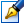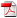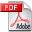##Boundary singularly perturbed problems for the evolutionary differential equations

 Author: Andrei Perjan Degree: doctor habilitat of physics and mathematics Speciality: 01.01.02 - Differential equations Year: 2009 Institution: Moldova State University Scientific council: DH 30-01.01.02-27.03.08 Moldova State University

### Status

The thesis was presented on the 5 December, 2008
Approved by NCAA on the 26 February, 2009

### Abstract0.37 Mb / in romanian

### Thesis

CZU 517.9561.27 Mb / in romanian
244 pages

### Keywords

singular perturbations, boundary layer, boundary layer function, symmetric systems of first order linear differential equations, differential equations in Hilbert spaces, a priori estimates, Sine-Gordon equation, Klein-Gordon equation.

### Summary

In the thesis we investigate the singularly perturbed problems for the systems of differential equations of the first order, and also for the second order semilinear differential equations in Hilbert spaces.

Theorems on existence of singular limits of solutions to the singularly perturbed Cauchy problem for systems of nonlinear differential equations in Hilbert spaces, and also for semilinear differential equations of the second order with lipschitzian, or local lipschitzian and monotone nonlinearities are established. Also, we proved theorems on the approximations of the solutions to the Cauchy problem of the first order semilinear equations in Hilbert spaces with lipschitzian, or local lipschitzian and monotone nonlinearities by solutions to the singularly perturbed Cauchy problem for the second order equations.

The thesis is written in Romanian.